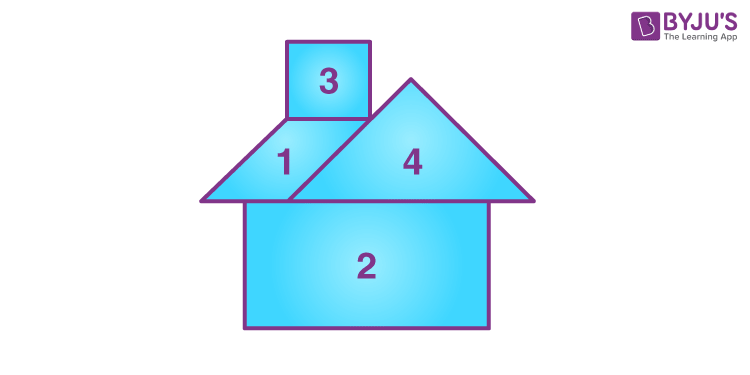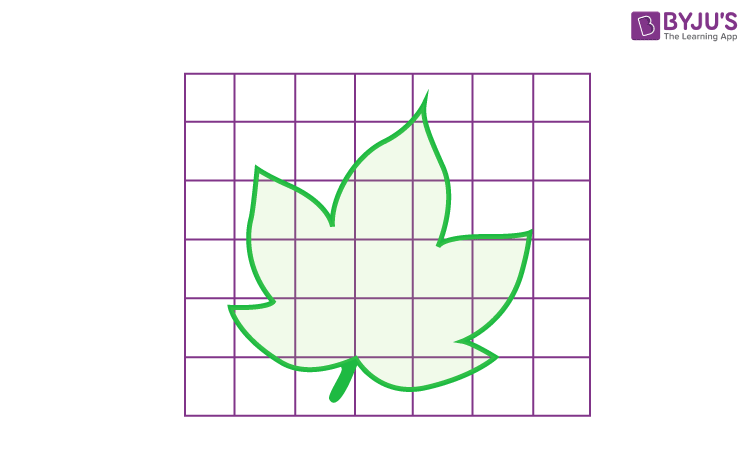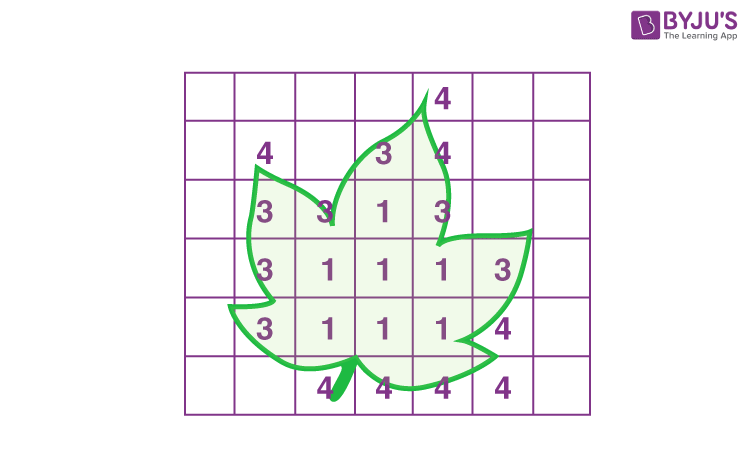Checkout JEE MAINS 2022 Question Paper Analysis : Checkout JEE MAINS 2022 Question Paper Analysis :

# Area of Irregular Shapes

Before learning about the area of irregular shapes, let’s recall what irregular shapes are and the various irregular shapes around us. Irregular shapes are those shapes that do not have equal sides and angles. A kite, leaf, flower, etc., are examples of irregular shapes around us. The area of these types of irregular shapes can be determined by dividing the shape into small regular polygons. In this article, you will learn the methods of finding the area of irregular shapes, along with the graphical illustration.

## What is the Area of Irregular Shapes?

As mentioned above, irregular shapes are those shapes that do not have equal sides or equal angles. The area of an irregular shape is the entire region covered by that shape on a two-dimensional plane. To find the area of an irregular shape, it can be divided into multiple familiar shapes, such as triangles, squares, and rectangles. Then, we can get the total area by adding the area of those smaller shapes. Also, the area of irregular shapes can be expressed in different units such as cm2, ft2, m2, etc.

Learn: Area

## How to Find the Area of Irregular Shapes?

Finding the area of irregular shapes is not as easy as it is for regular shapes or polygons. However, there are different methods to estimate the area of any irregular shape. Here, you will learn two important methods of finding the area of given irregular shapes. They are:

• Dividing the shape into possible regular polygons
• Using the graph paper

Let’s learn how we can estimate the area of irregular shapes using the decomposition of the shape into smaller polygons.

### Calculating the Area using Decomposition of an Irregular Shape

Consider an irregular shape as given below:The area of this irregular shape cannot be calculated using a single formula. Thus, we need to decompose the shape into possible simple polygons such as triangles, squares, and so on. This can be done as shown below:Therefore, the decomposed shape contains a parallelogram, rectangle, square and triangle. So, the area of the above irregular shape can be calculated as:

Area of the shape = Area of parallelogram + Area of rectangle + Area of square + Area of triangle

### Area of Irregular Shapes Using Graph Paper

Let’s learn how to find the area of irregular shapes using graph paper with the help of an example.

If the shape is given on graph paper, we can directly count the number of squares covered by that shape. Otherwise, we need to draw the graph lines or square grids over the given irregular shape.

Go through the example given below to understand this method of finding the area of an irregular shape in a better way.

Example of Area of Irregular Shapes by Counting Squares

Question:

Find the area of the shape in square units.Solution:

Let us count the squares that are completely covered, half covered and so on. Also, these can be marked as shown below:Now, we need to write the number of squares that are covered by the shape.

 Number assigned for covered portions Portions of squares covered Total 1 Fully covered squares 7 2 Half covered squares 0 3 More than half covered squares 7 4 Less than half covered squares 8

For fully covered square grids, we can take 1 square unit for each.

For half-covered squares, we should assign ½ square units for each.

If the square is covered more than half the portion, then assign 1 square unit for each such portion.

If the square grid is covered less than half, then assign 0 square units for each such portion.

Therefore, the area of the given shape = 7(1) + 0(½) + 7(1) + 8(0)

= 7 + 0 + 7 + 0

= 14

Hence, the area of the given irregular shape is 14 square units.

Similarly, we can find the area of irregular shapes given on graph paper. We can also draw the square grids, if it is not given on the graph paper.